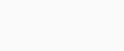# Queues

Difficulty
Easy 25%

Contenus

## Waiting lines

A queue (or queues) can be described as follows: clients (men, tasks, messages, etc.) arrive for a service, wait in a queue if they cannot be taken care of immediately , and leave after having had the service.

The model, originally created by Erlang for the 1909 telephone system, is used to model traffic management, planning and sizing of infrastructure and machining.

## Kendall's notation

A queue has 6 criteria noted by: T / X / C / K / P / Z

• T: the probability distribution of the inter-arrival time. Customers can leave if they arrive when the line is full. T can take the following values
• M for Markovian process / exponential law
• G for the general law
• D deterministic law
• E (k) for Erlang's law
• etc.

• X: the probability distribution of the service time. A client is taken directly by a free server, once the service is completed the client leaves. The distribution is represented by the same symbols as in T.
• C: the number of servers. In single queues, the servers all have the same uptime probability distribution.

• K: the capacity of the queue. If the queue is a finite number, the customer is lost when the queue is full. Servers count towards the capacity of the queue (1 per server).

• P: the size of the population. The size of the population is finite or infinite. In the case of a finite population, the customer arrival rate is a function of the number of customers in the system.
• Z: discipline of service
• FCFS / FIFO (first come first served / first in first out): first come first served
• LCFC / FILO (last come first served / first in last out): last come first served / first come last served
• RANDOM: random customer service in the waiting list
• HL (hold in line): if an "important" customer arrives, he takes the first place in the queue (depending on the "importance" of the first customers)
• PR (preemption): if an "important" customer arrives, he is served directly and the "less important" customer leaves the service to go in the queue
• PS (processor sharing): all customers are served at the same time with a speed inversely proportional to the number of customers
• etc.

A queue can be traversed by different classes of customers characterized by:

• Different arrival processes
• Different service times
• Different costs
• a scheduling in the queue according to their class

We will use the T / X / C notation when the queue is of infinite capacity, the size of the population is infinite and the service is FIFO discipline. This is equivalent to writing T / X / C / ∞ / ∞ / FIFO.

## Performance measures

The purpose of studying a queue is to calculate or estimate the performance of a system. This calculation is done in stationary mode in order to calculate: the speed X, the number of clients Q, the rate of use of the server U, the response time R. In general, the expectations of these parameters are calculated.

In the context of single queues, the system is ergodic. We then seek to calculate the stability of the system. Let's take the following:

• A (T): number of arrivals in the system between 0 and T
• D (T): number of system departures between 0 and T
• Xe (T) = A (T) / T: average inlet flow rate between 0 and T
• Xs (T) = D (T) / T: average outlet flow rate between 0 and T
• Q (T): average number of customers between 0 and T
• Rk: residence time of the kth customer arrived
•: average residence time between 0 and T

a queue is stable if and only if the limit when T approaches infinity of the average input rate is equal to the limit when T approaches infinity of the average output rate. In other words, when the limit as T approaches infinity of D (T) / A (T) = 1. In a stable queue, the number of customers remains finite.

Let Q be the average number of customers, R the average response time and X the average throughput, Little's law guaranteed in steady state for a stable system that Q=RX (one does not then take account of T any more).FRFRENRUES